International
Tables for
Crystallography
Volume D
Physical properties of crystals
Edited by A. Authier

International Tables for Crystallography (2006). Vol. D, ch. 2.2, pp. 305-307

## Section 2.2.14. Characterization of Bloch states

K. Schwarza*

aInstitut für Materialchemie, Technische Universität Wien, Getreidemarkt 9/165-TC, A-1060 Vienna, Austria
Correspondence e-mail: kschwarz@theochem.tuwein.ac.at

### 2.2.14. Characterization of Bloch states

| top | pdf |

The electronic structure of a solid is specified by energy bands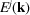and the corresponding wavefunctions, the Bloch functions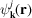. In order to characterize energy bands there are various schemes with quite different emphasis. The most important concepts are described below and are illustrated using selected examples in the following sections.

#### 2.2.14.1. Characterization by group theory

| top | pdf |

The energy bands are primarily characterized by the wavevectorin the first BZ that is associated with the translational symmetry according to (2.2.4.23). The star ofdetermines an irreducible basis provided that the functions of the star are symmetrized with respect to the small representations, as discussed in Section 2.2.6.Along symmetry lines in the BZ (e.g. fromalongtowards X in the BZ shown in Fig. 2.2.7.1), the corresponding group of thevector may show a group–subgroup relation, as for example forand. The corresponding irreducible representations can then be found by deduction (or by induction in the case of a group–supergroup relation). These concepts define the compatibility relations (Bouckaert et al., 1930; Bradley & Cracknell, 1972), which tell us how to connect energy bands. For example, the twofold degenerate representation(the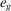symmetry in a cubic system) splits into the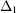and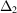manifold in thedirection, both of which are one-dimensional. The compatibility relations tell us how to connect bands. In addition, one can also find an orbital representation and thus knows from the group-theoretical analysis which orbitals belong to a certain energy band. This is very useful for interpretations.

#### 2.2.14.2. Energy regions

| top | pdf |

In chemistry and physics it is quite common to separate the electronic states of an atom into those from core and valence electrons, but sometimes this distinction is not well defined, as will be discussed in connection with the so-called semi-core states. For the sake of argument, let us discuss the situation in a solid using the concepts of the LAPW method, keeping in mind that very similar considerations hold for all other band-structure schemes.

A core state is characterized by a low-lying energy (i.e. with a large negative energy value with respect to the Fermi energy) and a corresponding wavefunction that is completely confined inside the sphere of the respective atom. Therefore there is effectively no overlap with the wavefunctions from neighbouring atoms and, consequently, the associated band width is practically zero.

The valence electrons occupy the highest states and have wavefunctions that strongly overlap with their counterparts at adjacent sites, leading to chemical bonding, large dispersion (i.e. a strong variation of the band energy with) and a significant band width.

The semi-core states are in between these two categories. For example, the 3s and 3p states of the 3d transition metals belong here. They are about 2–6 Ry (1 Ry = 13.6 eV) below the valence bands and have most of the wavefunctions inside their atomic spheres, but a small fraction (a few per cent) of the corresponding charge lies outside this sphere. This causes weak interactions with neighbouring atoms and a finite width of the corresponding energy bands.

Above the valence states are the unoccupied states, which often (e.g. in DFT or the HF method) require special attention.

#### 2.2.14.3. Decomposition according to wavefunctions

| top | pdf |

For interpreting chemical bonding or the physical origin of a given Bloch state at, a decomposition according to its wavefunction is extremely useful but always model-dependent. The charge density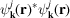corresponding to the Bloch state atcan be normalized to one per unit cell and is (in principle) an observable, while its decomposition depends on the model used. The following considerations are useful in this context:

 (1) Site-centred orbitals. In many band-structure methods, the Bloch functions are expressed as a linear combination of atomic orbitals (LCAO). These orbitals are centred at the various nuclei that constitute the solid. The linear-combination coefficients determine how much of a given orbital contributes to the wavefunction (Mulliken population analysis). (2) Spatially confined functions. In many schemes (LMTO, LAPW, KKR; see Section 2.2.11), atomic spheres are used in which the wavefunctions are described in terms of atomic-like orbitals. See, for example, the representation (2.2.12.1)in the LAPW method (Section 2.2.12), where inside the atomic sphere the wavefunction is written as an-like radial function times spherical harmonics (termed partial waves). The latter require a local coordinate system (Section 2.2.13) which need not to be the same as the global coordinate system of the unit cell. The reasons for choosing a special local coordinate system are twofold: one is a simplification due to the use of the point-group symmetry, and the other is the interpretation, as will be illustrated below for TiO2 in the rutile structure (see Section 2.2.16.2). (3) Orbital decomposition. In all cases in which-like orbitals are used (they do not require a local coordinate system) to construct the crystalline wavefunction, an-like decomposition can be done. This is true for both atom-centred orbitals and spatially confined partial waves. A corresponding decomposition can be done on the basis of partial electronic charges, as discussed below. A further decomposition into the m components can only be done in a local coordinate system with respect to which the spherical harmonics are defined. (4) Bonding character. As in a diatomic molecule with an orbital on atom A and another on atom B, we can form bonding and antibonding states by adding or subtracting the corresponding orbitals. The bonding interaction causes a lowering in energy with respect to the atomic state and corresponds to a constructive interference of the orbitals. For the antibonding state, the interaction raises the energy and leads to a change in sign of the wavefunction, causing a nodal plane that is perpendicular to the line connecting the nuclei. If the symmetry does not allow an interaction between two orbitals, a nonbonding state occurs. Analogous concepts can also be applied to solids. (5) Partial charges. The charge corresponding to a Bloch function of state– averaged over the star of– can be normalized to 1 in the unit cell. A corresponding decomposition of the charge can be done into partial electronic charges. This is illustrated first within the LAPW scheme. Using the resolution of the identity this 1 (unit charge) of each state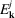can be spatially decomposed into the contribution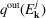from the region outside all atomic spheres (interstitial region II) and a sum over all atomic spheres (with superscript t) which contain the charges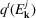(confined within atomic sphere t). The latter can be further decomposed into the partial-like charges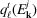, leading to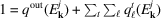. In a site-centred basis a similar decomposition can be done, but without the term. The interpretation, however, is different, as will be discussed for Cu (see Section 2.2.16). If the site symmetry (point group) permits, another partitioning according to m can be made, e.g. into the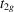andmanifold of the fivefold degenerate d orbitals in a octahedral ligand field. The latter scheme requires a local coordinate system in which the spherical harmonics are defined (see Section 2.2.13). In general, the proper m combinations are given by the irreducible representations corresponding to the site symmetry.

#### 2.2.14.4. Localized versus itinerant electrons

| top | pdf |

Simple metals with valence electrons originating from s- and p-type orbitals form wide bands which are approximately free-electron like (with a large band width W). Such a case corresponds to itinerant electrons that are delocalized and thus cause metallic conductivity.

The other extreme case is a system with 4f (and some 5f) electrons, such as the lanthanides. Although the orbital energies of these electrons are in the energy range of the valence electrons, they act more like core electrons and thus are tightly bound to the corresponding atomic site. Such electrons are termed localized, since they do not hop to neighbouring sites (controlled by a hopping parameter t) and thus do not contribute to metallic conductivity. Adding another of these electrons to a given site would increase the Coulomb repulsion U. A large U (i.e. t) prevents them from hopping.

There are – as usual – borderline cases (e.g. the late 3d transition-metal oxides) in which a delicate balance between t and U, the energy gain by delocalizing electrons and the Coulomb repulsion, determines whether a system is metallic or insulating. This problem of metal/insulator transitions is an active field of research of solid-state physics which shall not be discussed here.

In one example, however, the dual role of f electrons is illustrated for the uranium atom using relativistic wavefunctions (with a large and a small component) characterized by the quantum numbers n,and j. Fig. 2.2.14.1shows the outermost lobe (the large component) of the electrons beyond the [Xe] core without the 4f and 5d core-like states. One can see the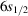,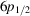and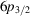(semi-core) electrons, and the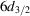and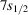(valence) electrons.Figure 2.2.14.1 | top | pdf |Relativistic radial wavefunctions (large component) of the uranium atom. Shown are the outer lobes of valence and semi-core states excluding the [Xe] core, and the 4f and 5d core states.

On the one hand, the radial wavefunction of the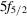orbital has its peak closer to the nucleus than the main lobes of the semi-core states,and, and thus demonstrates the core nature of these 5f electrons. On the other hand, theorbital decays (with distance) much less than the semi-core states and electrons in this orbital can thus also play the role of valence electrons, like electrons in theandorbitals. This dual role of the f electrons has been discussed, for example, by Schwarz & Herzig (1979).

#### 2.2.14.5. Spin polarization

| top | pdf |

In a non-fully-relativistic treatment, spin remains a good quantum number. Associated with the spin is a spin magnetic moment. If atoms have net magnetic moments they can couple in various orders in a solid. The simplest cases are the collinear spin alignments as found in ferromagnetic (FM) or antiferromagnetic (AF) systems with parallel (FM) and antiparallel (AF) moments on neighbouring sites. Ferrimagnets have opposite spin alignments but differ in the magnitude of their moments on neighbouring sites, leading to a finite net magnetization. These cases are characterized by the electronic structure of spin-up and spin-down electrons. More complicated spin structures (e.g. canted spins, spin spirals, spin glasses) often require a special treatment beyond simple spin-polarized calculations. In favourable cases, however, as in spin spirals, it is possible to formulate a generalized Bloch theorem and treat such systems by band theory (Sandratskii, 1990).

In a fully relativistic formalism, an additional orbital moment may occur. Note that the orientation of the total magnetic moment (spin and orbital moment) with respect to the crystal axis is only defined in a relativistic treatment including spin–orbit interactions. In a spin-polarized calculation without spin–orbit coupling this is not the case and only the relative orientation (majority-spin and minority-spin) is known. The magnetic structures may lead to a lowering of symmetry, a topic beyond this book.

#### 2.2.14.6. The density of states (DOS)

| top | pdf |

The density of states (DOS) is the number of one-electron states (in the HF method or DFT) per unit energy interval and per unit cell volume. It is better to start with the integral quantity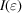, the number of states below a certain energy,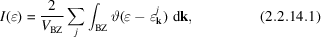where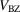is the volume of the BZ, the factor 2 accounts for the occupation with spin-up and spin-down electrons (in a non-spin-polarized case), and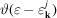is the step function, the value of which is 1 if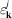is less thanand 0 otherwise. The sum overpoints has been replaced by an integral over the BZ, since thepoints are uniformly distributed. Both expressions, sum and integral, are used in different derivations or applications. The Fermi energy is defined by imposing that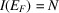, the number of (valence) electrons per unit cell.

The total DOS is defined as the energy derivative ofas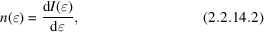with the normalization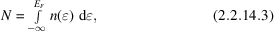where the integral is taken fromif all core states are included or from the bottom of the valence bands, often taken to be at zero. This defines the Fermi energy (note that the energy range must be consistent with N). In a bulk material, the origin of the energy scale is arbitrary and thus only relative energies are important. In a realistic case with a surface (i.e. a vacuum) one can take the potential at infinity as the energy zero, but this situation is not discussed here.

The total DOS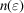can be decomposed into a partial (or projected) DOS by using information from the wavefunctions as described above in Section 2.2.14.3.If the charge corresponding to the wavefunction of an energy state is partitioned into contributions from the atoms, a site-projected DOS can be defined as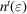, where the superscript t labels the atom t. These quantities can be further decomposed into-like contributions within each atom to give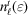. As discussed above for the partial charges, a further partitioning of the-like terms according to the site symmetry (point group) can be done (in certain cases) by taking the proper m combinations, e.g. theandmanifold of the fivefold degenerate d orbitals in a octahedral ligand field. The latter scheme requires a local coordinate system in which the spherical harmonics are defined (see Section 2.2.13). In this context all considerations as discussed above for the partial charges apply again. Note in particular the difference between site-centred and spatially decomposed wavefunctions, which affects the partition of the DOS into its wavefunction-dependent contributions. For example, in atomic sphere representations as in LAPW we have the decomposition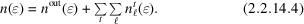In the case of spin-polarized calculations, one can also define a spin-projected DOS for spin-up and spin-down electrons.

### References

Bouckaert, L. P., Smoluchowski, R. & Wigner, E. (1930). Theory of Brillouin zones and symmetry properties of wavefunctions in crystals. Phys. Rev. 50, 58–67.
Bradley, C. J. & Cracknell, A. P. (1972). The mathematical theory of symmetry in solids. Oxford: Clarendon Press.
Sandratskii, L. M. (1990). Symmetry properties of electronic states of crystals with spiral magnetic order. Solid State Commun. 75, 527–529.
Schwarz, K. & Herzig, P. (1979). The sensitivity of partially filled f bands to configuration and relativistic effects. J. Phys. C Solid State Phys. 12, 2277–2288.